• 本文主要使用MATLAB产生一均匀分布的白噪声信号u(n)，画出其波形，并画出其直方图，检验其分布情况。 rand函数默认产生的是均值为0.5，幅度在0~1之间均有分布的伪随机数。 代码如下： %产生一均匀分布的白噪声...
本文主要使用MATLAB产生一均匀分布的白噪声信号u(n)，画出其波形，并画出其直方图，检验其分布情况。
rand函数默认产生的是均值为0.5，幅度在0~1之间均有分布的伪随机数。
代码如下：
%产生一均匀分布的白噪声信号u(n)，画出其波形，并检验其分布情况
clc
clear
close all
N=50000;          %u(n)的长度
u=rand(1,N);      %调用rand，得到均匀分布的伪随机数u(n)
u_mean=mean(u);   %求u(n)的均值，mean是MATLAB的m文件
power_u=var(u);   %求u(n)的方差
subplot(211)
plot(u(1:100));   %plot用来画连续曲线
grid on;          %给图形加网格
ylabel('u(n)')    %Y轴标题为u(n)
subplot(212)
hist(u,50);       %画u(n)的直方图,50是对u(n)取值范围(0~1)所分解的细胞数
grid on;
ylabel('histogram of u(n)');

运行图如下：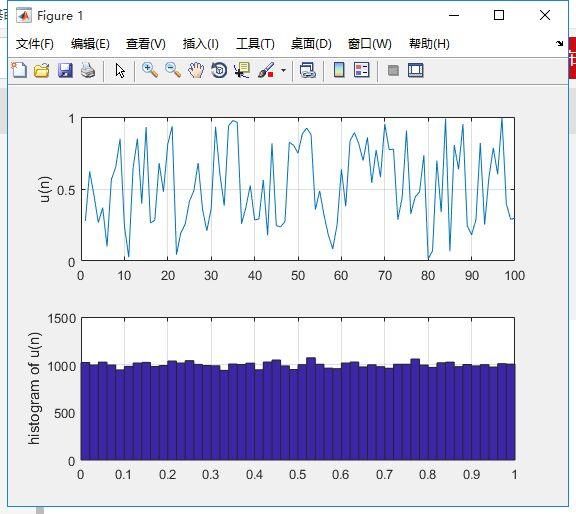展开全文• ## 【Matlab学习手记】添加随机噪声

万次阅读 多人点赞 2018-08-09 10:17:49
噪声是一种功率谱密度为常数的随机信号或随机过程，是功率谱密度在整个频域内均匀分布的噪声；相对的，其它不具有这一性质的噪声信号（功率谱密度不均匀分布）被称为有色噪声。 一个噪声过程所具有的频谱宽度远...
加性噪声和乘性噪声

白噪声是一种功率谱密度为常数的随机信号或随机过程，是功率谱密度在整个频域内均匀分布的噪声；相对的，其它不具有这一性质的噪声信号（功率谱密度不均匀分布）被称为有色噪声。

一个噪声过程所具有的频谱宽度远远大于它所作用系统的带宽。理想的白噪声具有无限带宽，因而其能量无限大，现实中不可能存在。实际上，我们常常将有限带宽的平整信号视为白噪声，因为这让我们在数学分析上更加方便。一般，只要一个噪声过程所具有的频谱宽度远远大于它所作用的系统的带宽，并且在该带宽中其频谱密度基本上可以作为常数来考虑，就可以把它作为白噪声来处理。例如，热噪声和散弹噪声在很宽的频率范围内具有均匀的功率谱密度，通常可以认为它们是白噪声。

加性噪声一般☞热噪声、散弹噪声等，它们与信号的关系是相加，不管有没有信号，噪声都存在；乘性噪声一般由信道不理想引起，它们与信号的关系是相乘，信号在它在，信号不在它就不在。一般通信中把加性随机性看成是系统的背景噪声，乘性随机性看成系统的时变性（如衰落或者多普勒）或者非线性所造成的。

Matlab中高斯白噪声函数

Matlab中产生高斯白噪声非常方便，可以直接调用两个函数：wgn和awgn，前者用于产生高斯白噪声，后者则用于在某一信号中加入高斯白噪声。

1. wgn产生高斯白噪声

y = wgn(m, n, p); % 产生一个m*n的高斯宝噪声，p以dBW为单位指定输出噪声的强度
y = wgn(m, n, p, imp); % 和上面的一样，除了欧姆（Ohm）为单位指定负载阻抗
y = wgn(m, n, p, imp, state); % 重置randn的状态
% 在数值变量后还可附加一些标志性参数
y = wgn(..., powertype); % 指定p的单位，‘dBW’，‘dBm’或‘linear’。线性强度（linear power）以瓦特（Watt）为单位
y = wgn(..., outputtype); % 指定输出类型，‘real’或‘complex’

2. awgn添加高斯白噪声

y = awgn(x, snr); % 在信号x中加入高斯白噪声，信噪比snr以dB为单位，如果x是复数，就加入复噪声，假定x的强度为0dBW
y = awgn(x, snr, sigpower); % 如果sigpower是数值，则其代表以dBW为单位的信号强度；如果sigpower为‘measured’，则函数将在加入噪声之前测定信号强度
y = awgn(x, snr, sigpower, state); % 重置randn的状态
y = awgn(..., powertype); % 指定snr和sigpower的单位

注释

1. 分贝（decibel，dB）表示相对功率或幅度电平的标准单位，换句话说，就是我们用来表示两个能量之间的差别的一种表示单位，它不是一个绝对单位。

2. 分贝瓦（dBW，dB Watt）☞以1W的输出功率为基准时，用分贝来测量的功率放大器的功率值。

randn产生高斯分布序列

y = randn(1, 2500);
y = y/std(y);
y = y - mean(y);
a = 0.01;
b = sqrt(0.81);
y = a + b*y;

上面的代码得到N(0.01, 0.81)的高斯分布序列。

如要产生均值为u，方差为σ^2的m*n的随机数矩阵，可以用 y = σ * randn(m, n) + u 得到。

如要产生[a, b]区间均匀分布的m*n的随机数矩阵，则可用 y = rand(m, n) * (b - a) + a 得到。

两点说明：1）rand产生的是[0, 1]上的均匀分布随机序列；2）randn产生均值为0，方差为1的高斯随机序列，即白噪声序列；也就是说，可以直接使用上面两个函数对原始信号添加噪声，如

y = x + rand(length(x), 1);
y = x + randn(length(x), 1);


展开全文randn rand
• 在进行控制系统仿真和随机过程仿真中，产生各种颜色的随机数是必...matlab自带了白噪声随机数生成算法，rand和randn,虽然其都是生成白噪声，但是rand产生的是均匀分布的噪声，而randn产生的是高斯分布的白噪声。这两...
在进行控制系统仿真和随机过程仿真中，产生各种颜色的随机数是必不可少的一步。考虑到实现的方便，这里使用matlab来进行仿真。通常的做法是先利用计算机自带的高斯白噪声生成函数生成白噪声，然后再利用算法产生所需要颜色的噪声，下面逐步进行介绍。matlab自带了白噪声随机数生成算法，rand和randn,虽然其都是生成白噪声，但是rand产生的是均匀分布的噪声，而randn产生的是高斯分布的白噪声。这两种方法产生的功率谱密度如下图所示，可以看到这两种方法生成的都是白噪声，仅是功率谱的幅值稍有不同。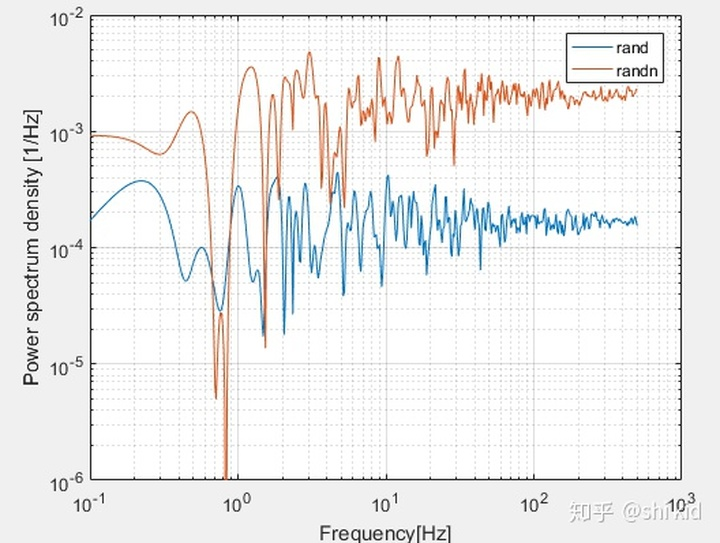使用直方图能更直观的观察到其差异：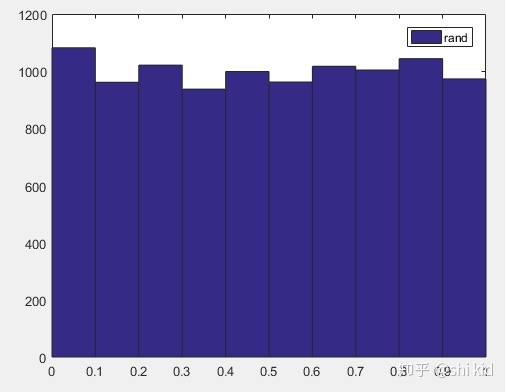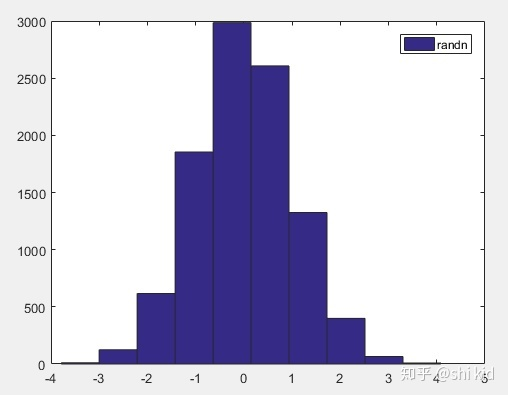在时钟产生的相位噪声里，噪声通常可以分为以下五种：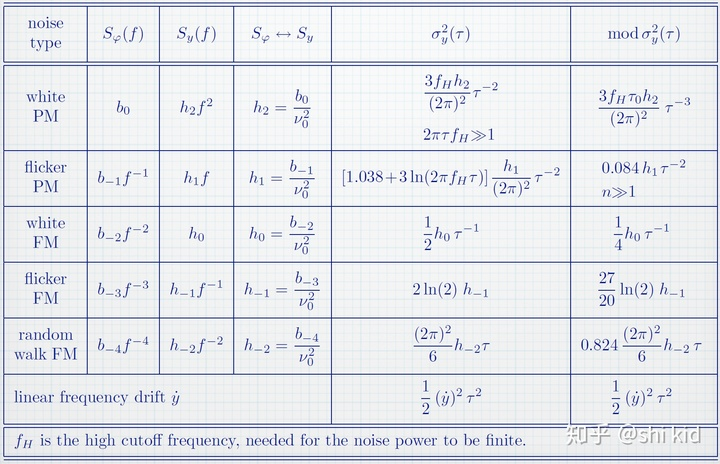因此，我们需要对着五种噪声进行建模。我们可以利用滤波器的概念来产生这些噪声。在数字信号中，我们知道，如果一个信号是白噪声，功率谱为S(f) = b0,即其功率谱密度与傅里叶频率无关，那么当这个信号通过一个1/f滚降的低通滤波器时，其输出将为So(f)=b0/f，即闪烁相位噪声。matlab代码如下N = 1e4;x = rand(N,1);xf = x;for ind = 2:N xf(ind) = xf(ind-1)+x(ind);endxf的功率谱密度如下图所示，其功率谱密度以滚降，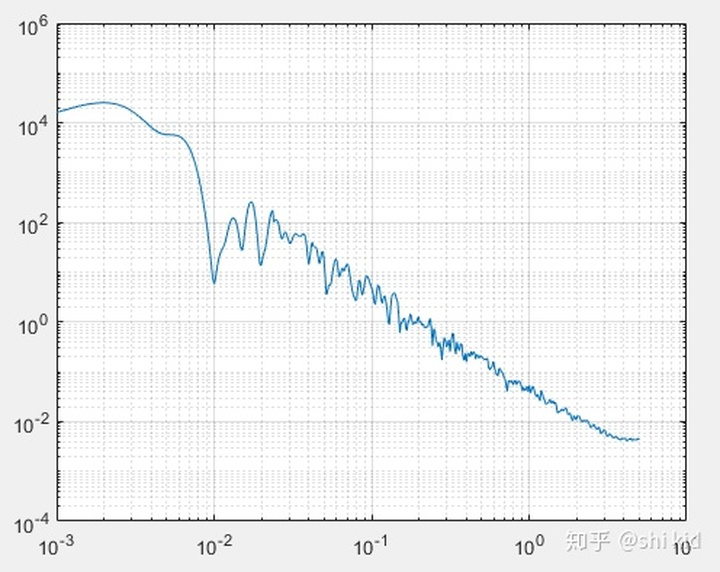同理，利用差分的方法，也可以得到功率谱密度正比于傅里叶频率的随机信号。
展开全文• 噪声就是标准均匀分布伪随机数列。 1.标准均匀分布函数，均值1/2，方差1/12； x1=1973; y=zeros(1,500); for i=1:500 x1=mod(91*x1,10^4); y(1,i)=x1/10000; end stem(y) xlabel('k');ylabel('NR');title('...
实现书本《随机控制》上关于生成高斯白噪声的方法。
白噪声就是标准均匀分布伪随机数列。
1.标准均匀分布函数，均值1/2，方差1/12；
 x1=1973;
y=zeros(1,500);
for i=1:500
x1=mod(91*x1,10^4);
y(1,i)=x1/10000;
end
stem(y)
xlabel('k');ylabel('NR');title('标准均匀分布伪随机序列');
mean(y)
var(y)
y1=zeros(1,4);n=1;
while n<=500
c=randperm(numel(y));b=y(c(1:12));
b1=b(1:6);b2=b(7:12);
d=sum(b1)-sum(b2);
y1(:,n)=d;
n=n+1;
end
figure(2)
stem(y1)
xlabel('n');ylabel('y1');title('由方法2生成的高斯白噪声');
mean(y1)
var(y1)



展开全文• randn(N,1)就是产生服从方差为1，均值为0 （ 即N(0,1) ）的Gaussian分布的NX1随机噪音矩阵，根据Gaussian...例：rand 生成均匀分布的伪随机数。分布在（0~1）之间 主要语法：rand(m,n)生成m行n列的均匀分布的伪随...randn
• rand产生的是[0，1]上的均匀分布的随机序列randn产生均值为0，方差为1的高斯随机序列，也就是白噪声序列 rand产生的是均匀分布白噪声序列randn产生的是正态分布的白噪声序列 MATLAB还提供了两个产生高斯白噪声的...
• （1）用计算机产生两个白噪声信号u1(t)和 u2(t)，u1(t)服从均匀分布，u2(t)服从高斯分布。 （2）用计算机产生一个随机信号x(t)=a(t)+u(t)，其中a(t)为确定性的正弦信号，u(t)为高斯分布的白噪声，u(t)的幅度大于a(t)...
• 所有频率具有相同能量密度的随机噪声称为白噪声。 2、均匀噪声、高斯白噪声、瑞利白噪声均匀噪声是幅度统计规律服从均匀分布而功率谱为常数的噪声 ②高斯白噪声是幅度统计规律服从高斯分布而功率谱为常数...
• （3） randperm(n)：产生1到n的均匀分布随机序列。 （4） normrnd(a,b,c,d)：产生均值为a、方差为b大小为cXd的 随机矩阵。 rand：返回一个在区间 (0,1) 内均匀分布的随机数。 rand(n)：生成0到1之间的n阶（ n×n ...
• ## matlab加随机噪音

千次阅读 2016-10-22 12:47:06
噪声(白杂讯)，是一种功率频谱密度为常数的随机信号或随机过程，是功率谱密度在整个频域内均匀分布的噪声。此信号在各个频段上的功率是一样的，由于白光是由各种频率（颜色）的单色光混合而成，因而此信号的这种...
• ## matlab生成随机矩阵

千次阅读 2014-03-11 16:45:29
原文地址：matlab生成随机矩阵作者：LFF_Coder 一. matlab里和随机数有关的函数： ...randperm(n):产生1到n的均匀分布随机序列 （4） normrnd(a,b,c,d)：产生均值为a、方差为b大小为cXd的随机矩阵 rand rand
• 一. matlab里和随机数有关的函数： ...（3） randperm(n):产生1到n的均匀分布随机序列 （4） normrnd(a,b,c,d)：产生均值为a、方差为b大小为cXd的随机矩阵 rand rand(n)：生成0到1之间的n阶随机数方阵 rand(m,n
• ## Matlab生成随机矩阵

千次阅读 2016-07-08 17:38:31
rand：产生幅度在0~1之间的伪随机数rand(n)：生成0到1之间的n阶随机数方阵rand(m,n)：生成0到1之间的m×n的随机数矩阵randn：产生均值为0、方差为1的高斯白噪声randn(n)：生成0均值，方差为1的n阶随机数方阵randn(m,...
• x=rand(m,n)%(0,1)均匀分布的白噪声序列 x=randn(m,n)正态分布白噪声序列 x=weibrnd(A,B,m,n)韦伯分布白噪声序列 %还有瑞利分布、伽马分布、指数分布等随机数产生函数 %相关正态随机序列的产生 a=0.8; sigma=2; ...
• 产生正弦波、均匀噪声、高斯白噪声图形。并分别将 两种噪声叠加在正弦波图形上，绘图 %1.正弦函数图形 及 叠加均匀噪声的图形 t=0:0.01:10; f=sin(t); y1=rand(size(f));%返回一个和f有相同尺寸的随机矩阵 y2=y1...信号处理 可视化
• 几个重要的概率密度函数 1.2.1 均匀分布 连续性随机变量X具有概率密度X：则称X在区间(a,b)上服从均匀分布，均匀分布的数字特征为：f(x)为概率密度分布函数，F(x)为分布函数，其分布函数为：MATLAB指令(1)R=unifrnd...
• % 【例8-8】利用rand函数产生服从（a-b）均匀分布的随机序列。 % 【例8-9】服从指数分布的热噪声随机序列的实现。 % 【例8-10】产生瑞利分布的热噪声。 % 【例8-7】利用randan函数产生高斯分布序列。 ...
• 噪声信号的产生在我们仿真雷达信号处理流程时，需要先仿真雷达的回波信号，同时为了更符合实际情况，我们还需要给雷达回波信号叠加上噪声或杂波。但由于噪声和杂波并不是确知信号，所以通过...下面给出Matlab中...
• 几个重要的概率密度函数 1.2.1 均匀分布 连续性随机变量X具有概率密度X：则称X在区间(a,b)上服从均匀分布，均匀分布的数字特征为：f(x)为概率密度分布函数，F(x)为分布函数，其分布函数为：MATLAB指令(1)R=unifrnd...
• Matlab 函数大全 信源函数 randerr 产生比特误差样本 randint 产生均匀分布的随机整数矩阵 randsrc 根据给定的数字表产生随机矩阵 wgn 产生高斯白噪声 信号分析函数 biterr 计算比特误差数和比特误差率 eyediagram ...
• . . . . .下载可编辑. 5.2 噪声和杂波的产生 在实际的雷达回波信号中不仅仅有...5.2.1 随机噪声 随机噪声有多种常见有概率密度函数服从高斯分布均匀分布指数分布以及分布的热噪声 1. 服从高斯Guass分布的热噪声
• 在统计信号处理中，往往会遇到等待处理的随机信号是非白色的，例如云雨、海浪、地物反射的杂乱回波等，它们的功率谱即使在信号通带内也非均匀分布。这样会给问题的解决带来困难。克服这一困难的措施之一是对色噪声...
• 第三章 随机信号与数字基带仿真 3.1 基本原理和实现示例 3.2 蒙特卡罗算法 第四章 模拟调制 MATLAB 实现 4.1 模拟调制 4.2 AM 调制解调的 MATLAB 实现 第五章 模拟信号的数字传输 5.1 脉冲编码调制 ...
• Matlab 通信仿真常用函数 信源函数 randerr 产生比特误差样本 randint 产生均匀分布的随机整数矩阵 randsrc 根据给定的数字表产生随机矩阵 wgn 产生高斯白噪声 信号分析函数 biterr 计算比特误差数和比特误差率 ...
• 产生均匀分布的随机序列、高斯白噪声随机序列、方波信号序列、三角波信号序列、正弦波信号序列，以及信噪比SNR为10dB的加性高斯白噪声正弦信号。 Ps=10; %信号功率10dBW Pn=1; %噪声功率0dBW f=100; %信号频率 fs=...
• 哈尔滨工程大学 研究生 随机过程实验 matlab源程序 均匀分布白噪声 正态分布 实验作业
• 信号的功率谱等于常数，其对应的随机过程称为白噪声，功率谱为常数意味着噪声在任何频率上都具有服从均匀分布的能量； 白噪声的一阶矩是常数，任何滞后的自相关系数均为0： R0=R1=...Rn=0R_{0}=R_{1}=...R_{n}=0R0​...
• 数字信号处理matlab练习【1】1.产生一个均匀分布的白噪声信号u(n),画出波形，并检验其分布2.产生一均匀分布、均值为0、功率为0.01...%使用rand函数产生均值为0.5，幅度在0-1之间的均匀分布的伪随机数 %以下将会调用...数字信号处理 rand函数 randn函数
• rand：产生均值为0.5、幅度在0~1之间的伪随机数，rand(n)：生成0到1之间的n阶随机数方阵，rand(m,n)：生成0到1之间的m×n的随机数矩阵 randn：产生均值为0、方差为1的高斯白噪声，使用方式同rand 注：rand是0-...# matlab随机均匀噪声matlab 订阅